Next: 7 Conclusions Up: 6 Quantum Diffusion II: Previous: 6.7 Numerical Evaluation of

# 6.8 Activated Tunnelling From Excited States

There also remains the possibility that the Mu tunnels rapidly from excited states, in which the barrier height and width would be reduced, so that the temperature dependence in the hop rate we measure is merely due to the temperature dependence of the probability of muonium occupying an excited state. We consider here a calculation of the vibrational frequency and the energy level separation of the harmonic oscillator modes of the muonium atom in the potential well defined by the xenon cage.

When several levels may be populated the total tunnelling rate from one potential well to another is the sum, assuming the states are populated according to the Boltzman distribution,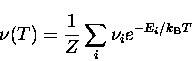(28)
where Ei is the energy of level i,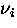the tunnelling rate from that level and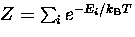is the partition function. In the FCC Xe lattice there are two sites, tetrahedral and octahedral, where the muonium atom may find space between the Xe atoms. We can calculate the shape of the potential well for these sites by summing the Xe-Mu pair potentials over the nearest Xe atoms at these sites, and from this obtain estimates of the energies of states in the harmonic well.

The Xe-H pair potential, at close distances r where the interaction is strongly repulsive, is approximated using the Born-Mayer potential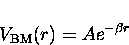(29)
with A=1023.3 eV and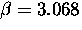Å. 

The Lennard-Jones (6,12) potential for the Xe-H pair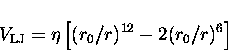(30)
with depth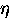=7.08 meV and equilibrium separation r0= 3.82 Å  is probably less accurate here since the equilibrium distance between each Xe atom and caged Mu atom is much shorter than the isolated Xe-Mu pair equilibrium separation of the Lennard-jones potential. (The lattice constant of solid Xe at 30 K is 6.197 Å.) If the Xe cage around the Mu atom does not relax, the Mu atom is 0.72 Å into the repulsive term of the potential, not near the minimum where the Lennard-Jones potential is more appropriate.

Within about 1Å of the equilibrium position of the Mu atom in the Xe cage this potential is very well approximated by a harmonic oscillator potential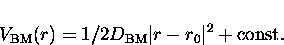(31)
where |r-r0| is the displacement from the equilibrium position r0 at the potential minimum in the well, and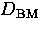is the restoring force constant. The energy levels of the quantum harmonic oscillator are spaced by an amount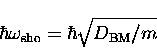(32)
for a particle of mass m in the well. Along the (111) direction in solid Xe the resulting constant is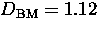eV/Å2 and this gives the Mu vibrational energy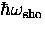= 0.202 eV, compared to the activation energy obtained from the Arrhenius plot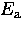= 0.048 eV. A similar calculation with the steeper Lennard-Jones potential and also with the Born-Mayer potential at the tetrahedral site, which allows less volume for the Mu atom, gives yet higher excitation energies.

If the Xe atoms are allowed to move away from the Mu atom, relaxing the strain imposed by the short Xe-Mu distance, the larger space for the Mu atom lowers the energy levels. The size of this shift can be estimated by iteratively minimizing the potential energy of Xe atoms and the Mu atom in a finite cluster of atoms. This calculation was done for a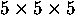unit cell cluster, with the Xe atoms at the surfaces of the cluster held at fixed positions of the undisturbed Xe lattice, and a Mu atom at the octahedral site of the central unit cell. After allowing all of the interior Xe atoms to find their new local potential minima, the new sites of the 6 nearest-neighbour Xe atoms that define the octahedral site are found to be displaced 0.20 Å away from the Mu atom. The potential of the Mu atom was then computed along the (111) direction through the octahedral site, with the lattice atoms now fixed, as shown in Fig. 6.31. (The heavy Xe atoms will not be able to follow the fast motion of the Mu atom.) Near the octahedral site the Mu potential is characterised by a force constant d = 0.485 eV/Å2, and is indicated by the dashed line. The first few energy levels of the resulting quantum harmonic oscillator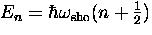are also shown, in which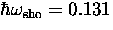eV, about 2.7 times larger than the measured activation energy Ea.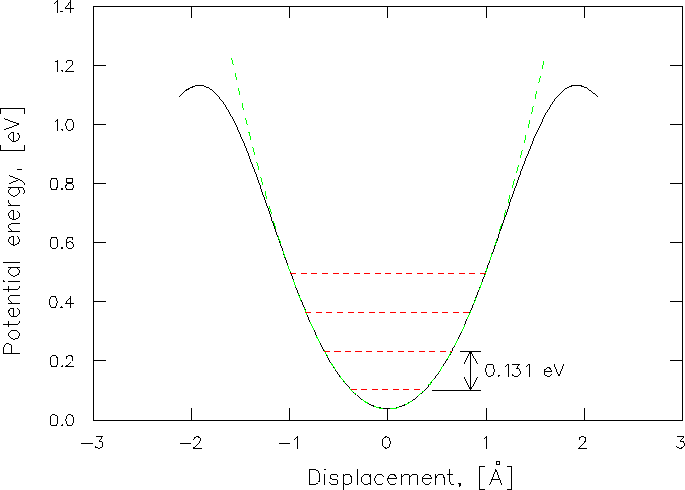We should conclude from this that classical thermal activation to excited states cannot be ruled out as being responsible for the temperature dependence of the Mu diffusion rate; the similarity of the calculated energy to the measured activation energy is simply too close for this question to be settled by this model. If muonium diffuses in solid Xe by quantum tunnelling, there is no evidence that barrier fluctuations play a role at any temperature, even under the most promising conditions for its detection.

At the temperatures being considered here there is a difficulty with any model involving coupling to phonons: the lack of knowledge of the frequency-dependence of the coupling parameters at frequencies that are no longer small compared to the Debye frequency. Further progress in understanding exactly how the interstitial couples to lattice excitations at frequencies where the solid is no longer Debye-like is clearly necessary if we are to fully understand the role of phonons in quantum diffusion. For example, direct measurement of the coupling constants as a function of wave vector (and polarization with respect to the lattice) are in principle possible by measuring the muonium hop rate while injecting phonons of a single wave vector at low temperture. This would have an enormous advantage over the indiscriminate thermal excitation of the entire phonon spectrum which has been the only method used thus far.Next: 7 Conclusions Up: 6 Quantum Diffusion II: Previous: 6.7 Numerical Evaluation of